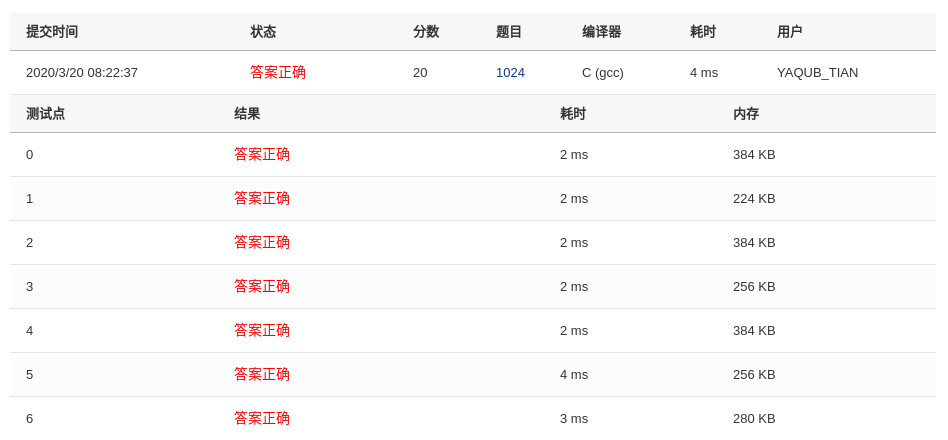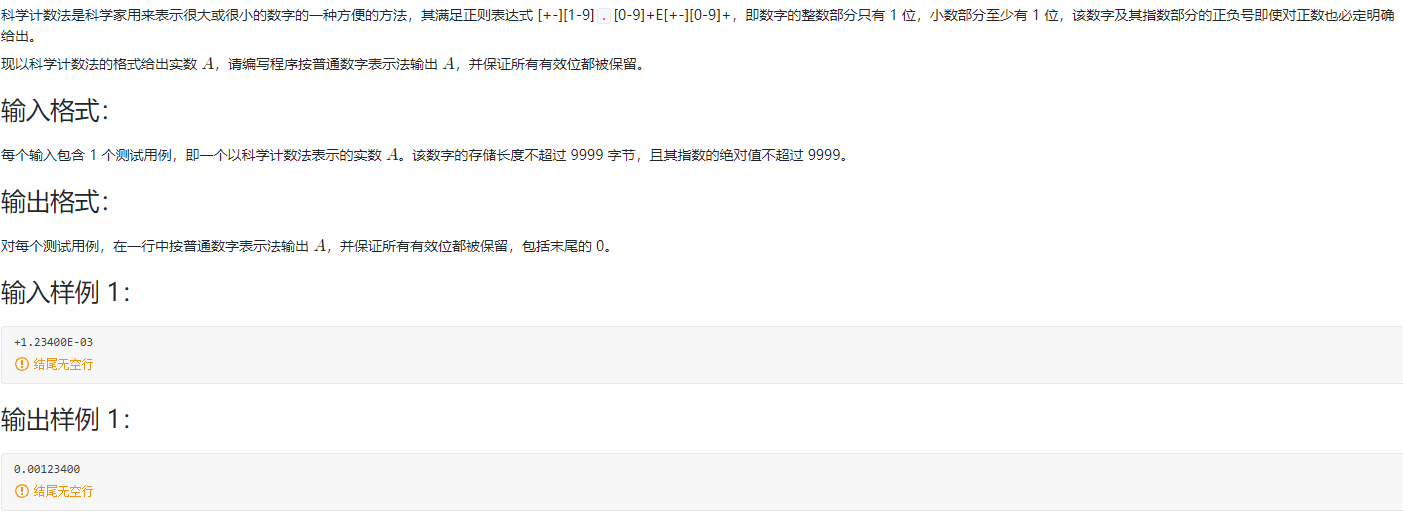• 【问题描述】 编写一个程序，将用科学计数法输入的一个数转换成小数表示的形式输出。该科学计数法表示的数字由以下几部分构成： 1.底数部分是一个小数，小数点前后必有数字，而且都为有效数字。即：小数点前只有一位...c++
• 科学计数法是科学家用来表示很大或很小的数字的一种方便的方法，其满足正则表达式 [±][1-9].[0-9]+E[±][0-9]+，即数字的整数部分只有 1 位，小数部分至少有 1 位，该数字及其指数部分的正负号即使对正数也必定明确...
前言
这个题目要考虑挺多小细节的（不过比起福尔摩斯那道还是hhh），我尽力了。。。
正文
题目
科学计数法是科学家用来表示很大或很小的数字的一种方便的方法，其满足正则表达式 [±][1-9].[0-9]+E[±][0-9]+，即数字的整数部分只有 1 位，小数部分至少有 1 位，该数字及其指数部分的正负号即使对正数也必定明确给出。
现以科学计数法的格式给出实数 A，请编写程序按普通数字表示法输出 A，并保证所有有效位都被保留。
输入格式：
每个输入包含 1 个测试用例，即一个以科学计数法表示的实数 A。该数字的存储长度不超过 9999 字节，且其指数的绝对值不超过 9999。

输出格式：
对每个测试用例，在一行中按普通数字表示法输出 A，并保证所有有效位都被保留，包括末尾的 0。

输入样例 1：
+1.23400E-03

输出样例 1：
0.00123400

输入样例 2：
-1.2E+10

输出样例 2：
-12000000000

代码
#include <stdio.h>
#include<string.h>
#define MAX 1000
int main(){
char s[MAX];
int flag=1,num[MAX];//指数符号和数组
int i,len=0,exp=0;
int j=0,n;
int iBegin=0,iEnd=0;//指数位起点和终点
scanf("%s",s);
n=strlen(s);//字符串长度
if(s=='-') //整数前的负号
printf("-");//是符号就直接输出
for(i=1;s[i]!='E';i++){
if(s[i]!='.'){
num[j++]=s[i]-'0';//存储E前的数字进入数组
len++;//数组长度
}
}
if(s[i+1]=='-'){// E后的符号，指数的正负
flag=-flag;
}
iBegin=i+2;//指数的第一位
iEnd=iBegin;
for(iEnd;iEnd<n;iEnd++){
exp=exp*10+(s[iEnd]-'0');//存放指数
}
/*第一种情况如果指数是0*/
if(exp==0){
for(j=0;j<len;j++)
printf("%d",num[j]);//输出数组
}
/*第二种情况如果指数是负数*/
if(flag==-1){
printf("0.");
while(exp-1){
printf("0");
exp--;
}
for(j=0;j<len;j++)
printf("%d",num[j]);//输出数组
}
/*第三种情况如果指数是正数*/
else if(flag==1){
if(exp<len-1) //第三种情况里的第一种情况：如果指数小于数组
{
for(j=0;j<len;j++){
printf("%d",num[j]);
if(j==exp){
printf(".");//输出小数点
}
}
}
else if(exp>=len-1){//第三种情况里的第二种情况：如果指数大于等于数组
for(j=0;j<len;j++){
printf("%d",num[j]);
}
while(exp-len+1){
printf("0");
exp--;
}
}
}
}

展开全文• 科学计数法向一般数字的转化，数组实现，以及浮点数实现法
• 即为符合C语言表示的科学记数表示。 输出： 该数据的双精度表示 说明： 输入数据的精度不高于小数点后50位。 输入数据时，在实数和幂之间有空格进行分隔，空格个数不定。 结果保留到小数点后8位，如不足8位...
对于非常大或者非常小的数据，我们通常用科学记数法来表示。例如在科技文献和电脑中经常遇到的 2.3×106 （计算机中的科学记数法表示为：2.3E6），或者 9.18×10-5 （科学记树法表示：9.18E-5）这种类型的数据。
输入：     用科学记数法表示的数据。即为符合C语言表示的科学记数法表示。
输出：     该数据的双精度表示
说明：     输入数据的精度不高于小数点后50位。     输入数据时，在实数和幂之间有空格进行分隔，空格个数不定。     结果保留到小数点后8位，如不足8位用0补足，超过8位则截断，不进行四舍五入的处理。
#include <stdio.h>
#include<math.h>
int main(){
int arr;int flen=0,blen=0,i=0,j=0,k=1,ci=0,e=1;char cc;
for(i=0;i<100;i++){//initialize
arr[i]=0;
}
i=0;
do{//输入小数点前的部分
cc=getchar();
ci=cc;
if((ci>=48)&&(ci<=57)){//判断为数字则存入数组
arr[i]=ci-48;i++;
flen++;//记录整数的位数
}
if(cc=='.'){//检测到小数点
break;
}
if(cc==' '){//检测到空格
break;
}
} while(1);
i=flen;
if('.'==cc){  //上个循环于小数点处结束，输入小数部分
do{
cc=getchar();
ci=cc;

if((ci>=48)&&(ci<=57)){//判断为数字，输入
arr[i++]=ci-48;
blen++;//记录小数的位数
}
if(cc==' '){//检测到空格，小数输入结束
break;
}
} while(1);
}

//end numbers input
do{
cc=getchar();
if((cc='e')||(cc='E')){
scanf(" %d",&e);
break;
}
}while(1);//Nothing wrong above
//printf("%d\n%d\n%d\n",flen,blen,e);
if(e>0){
for(j=0;j<flen+e;j++){
printf("%d",arr[j]);
}
printf(".");
for(k=flen+e;k<flen+e+8;k++){
printf("%d",arr[k]);
}
printf("\n");
return 0;
}
if(e==0){
for(j=0;j<flen;j++){
printf("%d",arr[j]);
}
printf(".");
for(k=flen;k<flen+8;k++){
printf("%d",arr[k]);
}
printf("\n");
return 0;
}
if(e<0){//power is nagetive
e=0-e;
if(e>=flen){//左移超出整数位数
printf("0.");
if((e-flen)<=8){
for(j=1;j<=e-flen;j++){
printf("0");
}}else{
for(j=1;j<=8;j++){
printf("0");}
}
for(k=0;k<=(7-e+flen);k++){
printf("%d",arr[k]);
}
printf("\n");
}else{//左移不超过整数位数
for(i=0;i<(flen-e);i++){
printf("%d",arr[i]);
}
printf(".");
for(i=flen-e;i<(flen-e+8);i++){
printf("%d",arr[i]);
}
printf("\n");	return 0;
}
}

}


展开全文开发语言 后端
• 要求输入科学计数法进行两者之间的加减乘除运算 并以科学计数法的形式输出 如： Values input: 0.25000e3 0.20000el Sum: 0.25200e3 Difference: 0.24800e3 Product: 0.50000e3 Quotient: 0.12500e3
• 这一位大佬的代码很厉害 这是地址 要保留所有有效数字，若是正数，前面的正号不输出。 #include <stdio.h> #define MAX_A 10000 char A[MAX_A];... /* E的位置，也是数字A除了指数部分外的末尾 */ ...
这一位大佬的代码很厉害 这是地址 要保留所有有效数字，若是正数，前面的正号不输出。#include <stdio.h>
#define	MAX_A	 10000
char A[MAX_A];
int pos_E;					/* E的位置，也是数字A除了指数部分外的末尾 */
int e;						/* 指数exponent */
char flag_E;				/* 指数的正负 */
int print_A();
int point_forward();		/* 小数点前移 */
int point_back();			/* 小数点后移 */
int print_m_n(int m, int n);
int print_0(int x);
/*************** 这个函数是读指数部分之前的部分 **************************/
/*************** 注意:小数点在数组A的第三个位置 **************************/
int c;

for(pos_E = 0; (c = getchar()) != 'E'; ++pos_E)
A[pos_E] = c;
}
/********************************* 读入指数 ******************************/
int i, j;
int c = 0;
int exp = {0};					/* 指数绝对值不超过四位 */

flag_E = getchar();
for(i = 0; (c = getchar()) != '\n'; ++i)
exp[i] = c - '0';
for(--i, j = 1; i >= 0; --i,j *= 10)
e += j*exp[i];
return 0;
}
/****************************** 输出A *************************************/
int print_A(){
if(A == '-')
putchar(A);
if(flag_E == '-')		/* 原小数点可能要前移  */
point_forward();
else					/* 原小数点可能要后移 */
point_back();
return 0;
}
/***************************** 小数点可能前移 ************************************/
int point_forward(){
if(e > 0 ){				/* 确实要前移 */
printf("0.");
print_0(--e);		/* 输出e--个零 */
printf("%c", A);	/* 再输出最后一个前移名额 */
print_m_n(3, pos_E);/* 输出其余的数 */
}else					/* e==0 输出 */
print_m_n(1, pos_E);
return 0;
}
/***************************** 小数点可能后移 ************************************/
int point_back(){
int i;

if(e == 0)
print_m_n(1, pos_E);
else if(e > 0 && e < (pos_E - 3)){/* 小数点确实要后移 */
printf("%c", A);	/* 它说保留所有有效位数字 */
print_m_n(3, 3+e);
printf(".");
print_m_n(3+e, pos_E);
}else if(e >= (pos_E - 3)){/* 没有小数点输出 */
printf("%c", A);
print_m_n(3, pos_E);
print_0(e - (pos_E - 3));
}
}
/************************** 输出A的下标从m到n的数 *******************************/
int print_m_n(int m, int n){
for(; m < n; ++m)
printf("%c", A[m]);
return 0;
}
/******************************* 输出x个零 *************************************/
int print_0(int x){
for(; x > 0; --x)
printf("0");
return 0;
}
int main(){
print_A();			/* 输出A */
return 0;
}


展开全文• i++)” 为什么要在这里加一个强制类型转换呢，我在测试的时候发现如果输入+1.2345E+3这里会一直循环 index是3，strlen(prime）是6，而3+2-6=-1，它应该不会进入这个循环啊，经过查询,strlen的返回值是unsigned int型...这个题真是学到了，先放一个自己的写法
#include<stdio.h>
#include<stdlib.h>
#include<string.h>
#include<math.h>
int main(){
char a,*prime;
scanf("%s",a);
if(a=='-')   //先打印一下符号
printf("-");
char *token=strtok(a+1,"E");   //strtok字符串分割函数，这里我就是想把小数和指数部分分割开来
prime=(char *)malloc(sizeof(char)*strlen(token));  //先给prime分配一下空间
strcpy(prime,token);   //让prime指向小数部分
token=strtok(NULL,"E");  //token此时指向指数部分
int index=atoi(token);   //index就是指数部分的数字,atoi是字符串转数字的函数

if(index<0)  //如果指数是负的
{
index=(-index)-1;  //先把指数变正方便操作
printf("0.");  //肯定是0.xxxx型的
while(index--)  //这里就相当于移动小数点了
printf("0");
for(int i=0;prime[i];i++)  //然后打印小数部分的数字就行了
if(prime[i]!='.')  //要排除.
printf("%c",prime[i]);
}
else if(index>=0)  //如果指针是非负的
{
for(int i=0;prime[i]&&i<index+2;i++)  //先打印小数点之前的数字，比如+1.2345E+3在这里就会输出1234
if(prime[i]!='.')
printf("%c",prime[i]);
int judge=0;

for(int i=0;i<(int)(index-strlen(prime)+2);i++){ //如果指数够大比如+1.23E+5就得输出123000了，所以需要打印0    这个为什么要加一个int强制转换类型后面再说
judge=1;  //判断它是不是这种指数够大的
printf("0");
}
if(judge==0&&(index-strlen(prime)+2)){  //如果指数不够大   判断(index-strlen(prime)+2)是为了防止+1.2E+1这样的输出12.
printf(".");  //先打印一个小数点
printf("%s",prime+index+2);  //再打印后面的数字
}

}

return 0;
}


后面学习了别人的方法，发现居然可以这样输入！
#include<stdio.h>
#include<stdlib.h>
#include<string.h>
#include<math.h>
int main(){
char a;
int index;
scanf("%[^E]E%d",a,&index);  //这样的输入方式就可以直接把小数和指数部分分开了，我只能说学到了
if(a=='-')
printf("-");
char *prime=a+1;
if(index<0)
{
index=(-index)-1;
printf("0.");
while(index--)
printf("0");
for(int i=0;prime[i];i++)
if(prime[i]!='.')
printf("%c",prime[i]);
}
else if(index>=0)
{
for(int i=0;prime[i]&&i<index+2;i++)
if(prime[i]!='.')
printf("%c",prime[i]);
int judge=0;

for(int i=0;i<(int)(index-strlen(prime)+2);i++){ //cnm
judge=1;
printf("0");
}
if(judge==0&&(index-strlen(prime)+2)){
printf(".");
printf("%s",prime+index+2);
}

}

return 0;
}


#include <stdio.h>
#include <stdlib.h>
#include <string.h>
int main()
{
int d,e,count=0;
char Dec;
scanf("%d.%[0-9]E%d",&d,Dec,&e);
printf("%s",d<0 ? "-" : "");
while( e++ < 0 )
printf("0%s",count++==0 ? "." : "");
e--;
printf("%d",abs(d));
for(int i=0 ; i<strlen(Dec) || i<e ; i++)
printf("%s%c",i==e && !count ? ".":"",i<strlen(Dec) ? Dec[i] : '0');
}


1.scanf的正则化输入，🐂🍺
2.后面的处理部分好像有点复杂，先插个眼后续改进
3.“for(int i=0;i<(int)(index-strlen(prime)+2);i++)” 为什么要在这里加一个强制类型转换呢，我在测试的时候发现如果输入+1.2345E+3这里会一直循环 index是3，strlen(prime）是6，而3+2-6=-1，它应该不会进入这个循环啊，经过查询,strlen的返回值是unsigned int型，而int 和 unsigned int计算时都会被转换为unsigned int型，而结果-1它现在是一个无符号整数，我们知道int型第一位是符号位,-1第一位是1，当他被当作无符号整数，第一位就不再是符号位了，这样-1就变成了一个很大的数，所以循环会一直进行，所以用strlen和整数参与运算的时候一定要注意
一个小测试
int main()
{
int a = -3;
unsigned int b = 1;
if(a+b>0)
printf("a+b>0\n");//这句话被打印
else
printf("a+b<0\n");
int z = a+b;
if(z > 0)
printf("z>0");
else
printf("z<0");//这句话被打印
}

展开全文• 变量不能作为e的指数?是的。doublea=3.5e2; doublea=3.5e0; doublea=3.5e-2; 只能是字面常量，而且必须是整数，正负0均可。。 doublea=3.5e2.1;//小数不行。。 doublea=3.5e;//没有指数也不行。...//变量也不...
• ## 科学计数法输出

千次阅读 2019-07-02 11:34:34
科学计数法的输出格式为：%e #include<iostream> using namespace std; int main() { double n=100000; printf("%e",n); return 0; } 输出： 1.000000e+005 表示1*10的5次方，即100000 #include<...c++ c
• 1024 科学计数法 (20 分) 科学计数法是科学家用来表示很大或很小的数字的一种方便的方法，...每个输入包含 1 个测试用例，即一个以科学计数法表示的实数 A。该数字的存储长度不超过 9999 字节，且其指数的绝对值不超过编程语言
• Sample Input 1 +1.23400E-03 Sample Output 1 ...思路： 字符串输入，先记录符号位，然后将E之前的数字转化成数字，将E后面的数字转化成幂的数。然后计算。 代码 #include<stdio.h> #include...
• 科学计数法是科学家用来表示很大或很小的数字的一种方便的方法，其...每个输入包含 1 个测试用例，即一个以科学计数法表示的实数A。该数字的存储长度不超过 9999 字节，且其指数的绝对值不超过 9999。 输出格式： ...开发语言 后端
• deal是科学计数法 转换函数 #include <iostream> #include <cstdio> #include <cstring> using namespace std; int n;//有效位数 string deal(string s, int & e)// e表示指数 可变的 初始...算法
• 科学计数法是科学家用来表示很大或很小的数字的一种方便的方法，其满足正则表达式 [±][1-9].[0-9]+E[±][0-9]+，即数字的整数部分只有 1 位，小数部分至少有 1 位，该数字及其指数部分的正负号即使对正数也必定明确...
• ## C++科学计数法

千次阅读 2020-08-31 06:21:29
float f1=3e2;//3*(10^2); f1结果为300; float f1 =3e-2;//3*(0.1^2); f2结果为0.03;
• 科学计数法是科学家用来表示很大或很小的数字的一种方便的方法，其满足正则表达式 [+-][1-9].[0-9]+E[+-][0-9]+，即数字的整数部分只有 1 位，小数部分至少有 1 位，该数字及其指数部分的正负号即使对正数也必定明确...
• 题目 科学计数法是科学家用来表示很大或很小的数字的一种方便的方法，其满足正则表达式[+-][1-9]"."[0-9]+E[+-][0-9]+，即数字的整数部分只有1位，小数...每个输入包含1个测试用例，即一个以科学计数法...
• 编写一个程序，将用小数表示的浮点数，转换成科学计数法的形式输出。输入的数据没有符号，小数点前后必有数字，且全为有效数据，即小数点后的末尾数字不为0；小数点前若只有一位数字，可以为0，否则小数点前的最高位...数据结构
• #define _CRT_SECURE_NO_WARNINGS #include<...struct SCINUM { // 科学计数法结构体 string valid_num; //有效位 int pow;//指数 SCINUM() { pow = 0; } }; SCINUM str2Sci(string num,int .
• timestamp=1608975458&ver=2790&signature=yyqyxpmtmy33R9J7OExoHd-3YNaYn9TM0FWkkkcydp2Z5XIJPNS5kkKGJT2FBDP8OT2C8u-PIEyOvbXQSY7N8b9vTp1cYs8LzSLUvBTiy4...C语言浮点型有三个类型：float、doublec
• C++字符串（string）实现科学计数法 在跟随《算法笔记》刷PAT时，多次遇到科学计数法相关的题目B1024，A1060,A1073做题过程中均耗费了大量的时间，因此对该类型题目做些总结 此类型题目属于字符串处理，string容器的...PAT 字符串处理 string容器 C++
• ## 科学计数法的输入

千次阅读 2015-05-27 17:23:23
1103: C二级辅导-阶乘数列 时间限制: 1 Sec 内存限制: 128 MB 提交: 178 解决: 63 [提交][状态][讨论版] ...科学计数法，保留两位小数。 输入 输出 样例输入 样例输出   1103: C二级辅
• 1024 科学计数法 (20 分) 科学计数法是科学家用来表示很大或很小的数字的一种方便的方法，...每个输入包含 1 个测试用例，即一个以科学计数法表示的实数 A。该数字的存储长度不超过 9999 字节，且其指数的绝对值不超过c++
• 使用以下方法，可正常显示。... * 当浮点型数据位数超过7位之后，数据变成科学计数法显示。用此方法可以使其正常显示。 * @param value * @return Sting */ public static String formatFloatNumber(doubl...浮点数 浮点数输出
• 将一个数转换成小数，输出结果为零 今天在处理数据的时候，有一个需求： 将一个整数，比如77000，单位纳秒（10^-9秒）换算成秒，然后保留10位小数。 代码： printf( "%d", (unsigned int(item2 &...
• 科学计数法C语言）_对方北方-CSDN博客 #include<stdio.h> #include<stdlib.h> int sort(char*a,int t,int p,int q); int main(){ char a; scanf("%s",a); int m,n,p,q;
• 如下，从文件中读取两个浮点数，并按照科学计数法打印出其和#include #include <stdlib.h>int main() { FILE *fp; double d1, d2; fp = fopen("string.in", "r"); if (fp == NULL) { printf("can not open file...
•  科学计数法是科学家用来表示很大或很小的数字的一种方便的方法，其满足正则表达式[+-][1-9]"."[0-9]+E[+-][0-9]+，即数字的整数部分只有1位，小数部分至少有1位，该数字及其指数部分的正负号即使对正数也必定明确...
• 用C++编程的时候，如果输出的数字太大，系统默认按科学计数法输出结果，这样的显示不是很直观，所以我们要改成平常用的十进制。 只需要在输出结果前面加上： cout.setf(ios_base::fixed,ios_base::floatfield); ...
• 1024 科学计数法 (20分) 科学计数法是科学家用来表示很大或很小的数字的一种方便的方法，...每个输入包含 1 个测试用例，即一个以科学计数法表示的实数 A。该数字的存储长度不超过 9999 字节，且其指数的绝对值不超过 9算法...

# c语言科学计数法输入c语言 订阅